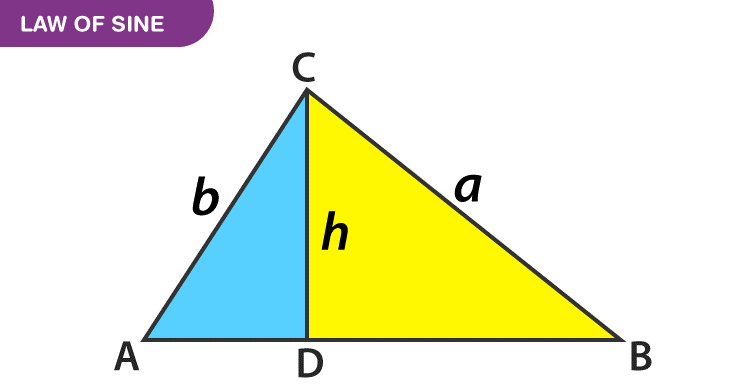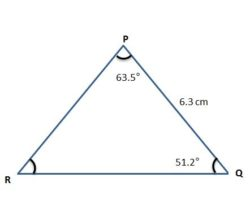# Law of Sines

Law of sines defines the ratio of sides of a triangle and their respective sine angles are equivalent to each other. The other names of the law of sines are sine law, sine rule and sine formula.

The law of sine is used to find the unknown angle or the side of an oblique triangle. The oblique triangle is defined as any triangle, which is not a right triangle. The law of sine should work with at least two angles and its respective side measurements at a time.

## Law of Sines Definition

In general, the law of sines is defined as the ratio of side length to the sine of the opposite angle. It holds for all the three sides of a triangle respective of their sides and angles.

 $$\begin{array}{l}\frac{a}{Sin A}=\frac{b}{Sin B}=\frac{c}{Sin C}\end{array}$$

The law of sine is explained in detail as follow:

In a triangle, side “a” divided by the sine of angle A is equal to the side “b” divided by the sine of angle B is equal to the side “c” divided by the sine of angle C.

##So, we use the Sine rule to find unknown lengths or angles of the triangle. It is also called as Sine Rule, Sine Law or Sine Formula. While finding the unknown angle of a triangle, the law of sines formula can be written as follows:

(Sin A/a) = (Sin B/b) = (Sin C/c)

In this case, the fraction is interchanged. It means that Sin A/a, instead of taking a/sin A.

## Law of Sines Proof

We need a right-angled triangle to prove the above as the trigonometric functions, which are mostly defined in terms of this type of triangle only.

Given: △ABC, AB = c, BC = a and AC = b.

Construction: Draw a perpendicular, CD ⊥ AB. Then CD = h is the height of the triangle. “h” separates the △ ABC in two right-angled triangles, △CDA and △CDB.

To Show: a / b = Sin A / Sin B

Proof: In the  △CDA,

Sin A= h/b

And in  △CDB,

Sin B = h/a

Therefore, Sin A / Sin B = (h / b) / (h / a)= a/b

And we proved it.

Similarly, we can prove, Sin B/ Sin C= b / c and so on for any pair of angles and their opposite sides.

## Sine Formula

The formulas used with respect to the law of sine are given below.

 a / Sin A= b/ Sin B= c / Sin C a: b: c = Sin A: Sin B: Sin C a / b = Sin A / Sin B b / c = Sin B / Sin C

It denotes that if we divide side a by the Sine of ∠A, it is equal to the division of side b by the Sine of∠ B and also equal to the division of side c by Sine of ∠C (Or) The sides of a triangle are to one another in the same ratio as the sines of their opposite angles.

Here, Sin A is a number and a is the length.

## Applications of Sine Law

The trigonometry ratios such as sine, cosine and tangent are primary functions that are used to find the unknown angles or sides of a right triangle. The applications of sine law are given below:

• It can be used to compute the other sides of a triangle when two angles and one side is given.
• When two sides and one non-included angle are given.

The sine law is used to find the unknown angle or unknown side.

As per the law, we know, if a, b and c are the lengths of three sides of a triangle and ∠A, ∠B and ∠C are the angles between the sides, then;

a/sin A = b/sin B = c/sin C

Now if suppose, we know the value of one of the sides, and the value of two angles, such as:

a = 7 cm, ∠A = 60°, ∠B = 45°

find b?

By the sine law, we know;

a/sin A = b/sin B

Now putting the values, we get;

7/sin 60° = b/sin 45°

7/(√3/2) = b/(1/√2)

14/√3 = √2 b

b = 14/(√3√2) = 14/√6

## Law of Sines Uses

The law of sines is generally used to find the unknown angle or side of a triangle. This law can be used if certain combinations of measurement of a triangle are given.

1. ASA Criteria: Given two angles and included side, to find the unknown side.
2. AAS Criteria: Given two angles and a non-included side, to find the unknown side.

These two criteria will provide a unique solution, as AAS and ASA methods are used to prove the congruence of triangles.

Ambiguous Case:

In an ambiguous case, if in a triangle two sides and the angle opposite to them are known, then there could be three possibilities:

• That there is no such triangle
• There are two different triangle
• Or there is exactly one such triangle

### Law of sines in Real life

• In real life, the law of sines is used in engineering, to measure the angle of tilt.
• It is used in astronomy, to measure the distance between planets or stars
• Also, the measurement of navigation is possible using the law of sines

## Law of sines and Cosines

Both the law of sines and cosines are used to find the unknown angle or an unknown side of a triangle. Let us counter the difference between the two laws.

 Law of sines Law of cosines It is used when we are given with: two angles and one side, or two sides and a non-included angle It is used when we are given with: three sides, or two sides and the included angle. If a, b and c are the sides and A, B and C are the angles of a triangle, then sine rule is given by: a/SinA = b/SinB = c/SinC If a, b and c are the sides and A, B and C are the angles of a triangle, then cosine rule is given by: a2 = b2 + c2 − 2bc cos A b2 = a2 + c2 − 2ac cos B c2 = a2 + b2 − 2ab cos C

## Solved Example

Question: Solve △PQR in which ∠ P = 63.5° and ∠Q = 51.2°  and r = 6.3 cm.Solution:

Let’s Calculate the third angle-

∠R = 180° – 63.5°- 51.2 °= 65.3°

Now, Let’s calculate the sides:

6.3/Sin 65.3 = p/Sin 63.5

p = (6.3 × Sin 63.5)/Sin 65.3

p= 6.21 cm approximately.

Similarly, 6.3/Sin 65.3 = q/Sin 51.2

q = (6.3 × Sin 51.2) / Sin 65.3

q= 5.40 cm

∠R= 65.3°

p=6.21 cm

q=5.40 cm

## Frequently Asked Questions on Law of sines

### What is meant by the law of sines?

The law of sine or the sine law states that the ratio of the side length of a triangle to the sine of the opposite angle, which is the same for all three sides. It is also known as the sine rule.

### Write down the sine rule.

If a, b, and c are the sides of a triangle, and A, B, and C are the angles, then the sine rule or the law of sine is given by
(a/sin A) = (b/sin B) = (c/ sin C)

### Why do we use the law of sines?

The law of sines is used to determine the unknown side of a triangle when two angles and sides are given.

### In which cases, can we use the law of sines?

Generally, the law of sines is used to solve the triangle, when we know two angles and one side or two angles and one included side. It means that the law of sines can be used when we have ASA (Angle-Side-Angle) or AAS (Angle-Angle-Side) criteria.

### Mention the other way to represent the sine rule formula.

The other way to represent the sine law or sine rule is a: b: c = Sin A: Sin B: Sin C.

Get started with Practicing more of trigonometric problems with BYJU’S – The Learning App.

Test your knowledge on Law Of Sines